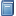Buchempfehlung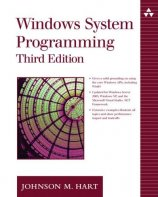Windows System Programming
Das Kompendium liefert viele interessante Informationen zur Windows-Programmierung auf Englisch. [Mehr Infos...]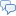FreeBASIC-Chat
Es sind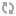Benutzer im FreeBASIC-Chat online.
(Stand:  )

# fb:porticula NoPaste

## TSNEX_FTP.bi

 Uploader: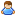ThePuppetMaster Datum/Zeit: 21.01.2008 21:29:40

```'##################################################################################################### '##################################################################################################### ' TSNE_V2 - TCP Socket Networking [Eventing] Version: 2 ' Erweiterungs-Bibliothek '##################################################################################################### '##################################################################################################### ' 2008 By.: /_\ DeltaLab's - Deutschland ' Autor: Martin Wiemann '##################################################################################################### '############################################################################################################## #include once "TSNE_V2.bi" #include once "TSNEX_helper.bi" '############################################################################################################## Dim Shared TSNEX_FTP as UInteger Dim Shared TSNEX_FTP_DataRX as String Dim Shared TSNEX_FTP_What as Long Dim Shared TSNEX_FTP_Local as String Dim Shared TSNEX_FTP_FID as Integer Dim Shared TSNEX_FTP_AKP as ULongInt Dim Shared TSNEX_FTP_Kill as UByte Dim Shared TSNEX_FTP2 as UInteger Dim Shared TSNEX_FTP2_DataRX as String Dim Shared TSNEX_FTP2_DataTX as String Dim Shared TSNEX_Path as String Dim Shared TSNEX_File as String Dim Shared TSNEX_Type as String Dim Shared TSNEX_User as String Dim Shared TSNEX_Pass as String Dim Shared TSNEX_BV as Long '############################################################################################################## Declare Function    TSNEX_FTP_List          (V_URL as String, B_FolderD() as String, ByRef B_FolderC as ULong, B_FileD() as String, ByRef B_FileC as ULong) as Long Declare Function    TSNEX_FTP_Download      (V_URL as String, V_LocalTarget as String, V_KillExist as UByte = 0) as Long Declare Function    TSNEX_FTP_Upload        (V_URL as String, V_LocalSource as String, V_KillExist as UByte = 0) as Long '############################################################################################################## Sub TSNE_FTP2_Disconnected(ByVal V_TSNEID as UInteger) End Sub '-------------------------------------------------------------------------------------------------------------- Sub TSNE_FTP2_Connected(ByVal V_TSNEID as UInteger) Select case TSNEX_FTP_What     case 3         Dim D as String         Dim X as ULongInt         Dim XFL as ULongInt         Dim XBSize as Long = 8092         XFL = Lof(TSNEX_FTP_FID)         For X = 1 to XFL step XBSize             D = Space(XBSize)             If (X + XBSize) > XFL Then D = Space(XFL - X + 1)             Get #TSNEX_FTP_AKP, X, D             TSNE_Data_Send(V_TSNEID, D)         Next         TSNE_Disconnect(V_TSNEID) End select End Sub '-------------------------------------------------------------------------------------------------------------- Sub TSNE_FTP2_NewData(ByVal V_TSNEID as UInteger, ByRef V_Data as String) Select case TSNEX_FTP_What     case 1: TSNEX_FTP2_DataRX += V_Data     case 2: Put #TSNEX_FTP_FID, TSNEX_FTP_AKP, V_Data: TSNEX_FTP_AKP += Len(V_Data) End select End Sub '-------------------------------------------------------------------------------------------------------------- Sub TSNE_FTP_NewData(ByVal V_TSNEID as UInteger, ByRef V_Data as String) TSNEX_FTP_DataRX += V_Data Dim XFBCRLF as String = Chr(13, 10) Dim T as String Dim T1 as String Dim T2 as UShort Dim XCMD as UInteger Dim XPos as UInteger Dim BV as Long Do     XPos = InStr(1, TSNEX_FTP_DataRX, XFBCRLF)     If XPos > 0 Then         T = Mid(TSNEX_FTP_DataRX, 1, XPos - 1): TSNEX_FTP_DataRX = Mid(TSNEX_FTP_DataRX, XPos + 2)         XCMD = Val(Mid(T, 1, 3)): T = Mid(T, 5)         Select Case XCMD             case 150: TSNE_Data_Send(V_TSNEID, "PASV" & XFBCRLF)             case 200                 Select case TSNEX_FTP_What                     case 1: TSNE_Data_Send(V_TSNEID, "LIST" & XFBCRLF)                     case 2: TSNE_Data_Send(V_TSNEID, "RETR " & TSNEX_File & TSNEX_Type & XFBCRLF)                     case 3: TSNE_Data_Send(V_TSNEID, "STOR " & TSNEX_File & TSNEX_Type & XFBCRLF)                 End select             case 220                 If TSNEX_User = "" Then                 Else: TSNE_Data_Send(V_TSNEID, "USER " & TSNEX_User & XFBCRLF)                 End If             case 221'Quit             case 226: TSNEX_BV = 0: TSNE_Disconnect(V_TSNEID): Exit Sub             case 227                 If TSNEX_FTP2 = 0 Then                     XPos = InStr(1, T, "(")                     If XPos <= 0 then TSNEX_BV = 7: TSNE_Disconnect(V_TSNEID): Exit Sub                     T = Mid(T, XPos + 1)                     XPos = InStr(1, T, ")")                     If XPos <= 0 then TSNEX_BV = 7: TSNE_Disconnect(V_TSNEID): Exit Sub                     T = Mid(T, 1, XPos - 1)                     For BV = 1 to Len(T)                         XPos = InStr(1, T, ",")                         If XPos > 0 Then                             Select Case BV                                 case 1 to 4: T1 += Mid(T, 1, XPos - 1) & ".": T = Mid(T, XPos + 1)                                 case else: T2 = (256 * Val(Mid(T, 1, XPos - 1))) + Val(Mid(T, XPos + 1)): Exit For                             End Select                         End If                     Next                     If (T1 = "") or (T2 <= 0) Then TSNEX_BV = 7: TSNE_Disconnect(V_TSNEID): Exit Sub                     T1 = Left(T1, Len(T1) - 1)                     BV = TSNE_Create_Client(TSNEX_FTP2, T1, T2, 0, @TSNE_FTP2_Connected, @TSNE_FTP2_NewData)                 End if             case 230: TSNE_Data_Send(V_TSNEID, "CWD " & TSNEX_Path & XFBCRLF)             case 250: TSNE_Data_Send(V_TSNEID, "TYPE A" & XFBCRLF)             case 331: TSNE_Data_Send(V_TSNEID, "PASS " & TSNEX_Pass & XFBCRLF)             case 530: TSNEX_BV = 6: TSNE_Disconnect(V_TSNEID): Exit Sub             case 550: TSNEX_BV = 13: TSNE_Disconnect(V_TSNEID): Exit Sub             case else: TSNE_Disconnect(V_TSNEID): Exit Sub         End Select     Else: Exit Do     End If Loop End Sub '############################################################################################################## Function TSNEX_FTP_List(V_URL as String, B_FolderD() as String, ByRef B_FolderC as ULong, B_FileD() as String, ByRef B_FileC as ULong) as Long Dim XProt as String Dim XHost as String Dim XPort as UShort Dim BV as Long Dim XFBCRLF as String = Chr(13, 10) BV = URL_Split(V_URL, XProt, XHost, XPort, TSNEX_Path, TSNEX_File, TSNEX_Type, TSNEX_User, TSNEX_Pass) If BV <> 0 Then Return BV If LCase(XProt) <> "ftp" then Return 2 If XPort = 0 Then XPort = 21 If TSNEX_Pass = "" Then TSNEX_Pass = "TSNEX@FTP.Ext" TSNEX_FTP_What = 1 TSNEX_FTP_DataRX = "" TSNEX_FTP_Local = "" TSNEX_FTP_AKP = ValLng("1") TSNEX_BV = -1 BV = TSNE_Create_Client(TSNEX_FTP, XHost, XPort, 0, 0, @TSNE_FTP_NewData) If BV < 0 Then Return BV TSNE_WaitClose(TSNEX_FTP) TSNE_Disconnect(TSNEX_FTP2) TSNE_WaitClose(TSNEX_FTP2) TSNEX_FTP = 0 TSNEX_FTP2 = 0 Dim XPos as UInteger Dim T as String Do     XPos = InStr(1, TSNEX_FTP2_DataRX, XFBCRLF)     If XPos > 0 Then         T = Mid(TSNEX_FTP2_DataRX, 1, XPos - 1)         TSNEX_FTP2_DataRX = Mid(TSNEX_FTP2_DataRX, XPos + 2)     Else: T = TSNEX_FTP2_DataRX: TSNEX_FTP2_DataRX = ""     End If     If T <> "" Then         If Left(T, 1) = "-" Then             B_FileC += 1             Redim Preserve B_FileD(B_FileC) as String             B_FileD(B_FileC) = Mid(T, 56)         Else             B_FolderC += 1             Redim Preserve B_FolderD(B_FolderC) as String             B_FolderD(B_FolderC) = Mid(T, 56)         End If     End If     If TSNEX_FTP2_DataRX = "" Then Exit Do Loop Return TSNEX_BV End Function '############################################################################################################## Function TSNEX_FTP_Download(V_URL as String, V_LocalTarget as String, V_KillExist as UByte = 0) as Long Dim XProt as String Dim XHost as String Dim XPort as UShort Dim BV as Long Dim XFBCRLF as String = Chr(13, 10) BV = URL_Split(V_URL, XProt, XHost, XPort, TSNEX_Path, TSNEX_File, TSNEX_Type, TSNEX_User, TSNEX_Pass) If BV <> 0 Then Return BV If LCase(XProt) <> "ftp" then Return 2 If TSNEX_File = "" Then Return 9 If TSNEX_Type <> "" Then TSNEX_Type = "." & TSNEX_Type If XPort = 0 Then XPort = 21 If TSNEX_Pass = "" Then TSNEX_Pass = "TSNEX@FTP.Ext" TSNEX_FTP_What = 2 TSNEX_FTP_DataRX = "" TSNEX_FTP_Local = V_LocalTarget TSNEX_FTP_AKP = ValLng("1") TSNEX_BV = -1 If (TSNEX_FTP_Local = "") or (Right(TSNEX_FTP_Local, 1) = TSNEX_Seperator) Then TSNEX_FTP_Local += TSNEX_File & TSNEX_Type If Dir(TSNEX_FTP_Local, -1) <> "" Then     If V_KillExist = 0 Then Return 8     If Kill(TSNEX_FTP_Local) <> 0 Then Return 11 End If TSNEX_FTP_FID = FreeFile If Open(TSNEX_FTP_Local For Binary as #TSNEX_FTP_FID) <> 0 Then Return 10 BV = TSNE_Create_Client(TSNEX_FTP, XHost, XPort, 0, 0, @TSNE_FTP_NewData) If BV < 0 Then Return BV TSNE_WaitClose(TSNEX_FTP) TSNE_Disconnect(TSNEX_FTP2) TSNE_WaitClose(TSNEX_FTP2) TSNEX_FTP = 0 TSNEX_FTP2 = 0 If TSNEX_FTP_FID <> 0 Then Close #TSNEX_FTP_FID Return TSNEX_BV End Function '############################################################################################################## Function TSNEX_FTP_Upload(V_URL as String, V_LocalSource as String, V_KillExist as UByte = 0) as Long Return -1 If V_LocalSource = "" Then Return 12 Dim XProt as String Dim XHost as String Dim XPort as UShort Dim BV as Long Dim XPos as UInteger Dim XFBCRLF as String = Chr(13, 10) BV = URL_Split(V_URL, XProt, XHost, XPort, TSNEX_Path, TSNEX_File, TSNEX_Type, TSNEX_User, TSNEX_Pass) If BV <> 0 Then Return BV If LCase(XProt) <> "ftp" then Return 2 If TSNEX_File = "" Then     XPos = InStrRev(V_LocalSource, TSNEX_Seperator)     If XPos > 0 Then         TSNEX_File = Mid(V_LocalSource, XPos + 1)     Else: TSNEX_File = V_LocalSource     End if     XPos = InStr(1, V_LocalSource, ".")     If XPos > 0 Then TSNEX_Type = Mid(TSNEX_File, XPos + 1): TSNEX_File = Mid(TSNEX_File, 1, XPos - 1) End If If TSNEX_Type <> "" Then TSNEX_Type = "." & TSNEX_Type If XPort = 0 Then XPort = 21 If TSNEX_Pass = "" Then TSNEX_Pass = "TSNEX@FTP.Ext" TSNEX_FTP_What = 3 TSNEX_FTP_DataRX = "" TSNEX_FTP_Local = "" TSNEX_FTP_AKP = ValLng("1") TSNEX_BV = -1 TSNEX_FTP_Kill = V_KillExist TSNEX_FTP_FID = FreeFile If Open(V_LocalSource For Binary as #TSNEX_FTP_FID) <> 0 Then Return 10 BV = TSNE_Create_Client(TSNEX_FTP, XHost, XPort, 0, 0, @TSNE_FTP_NewData) If BV < 0 Then Return BV TSNE_WaitClose(TSNEX_FTP) TSNE_Disconnect(TSNEX_FTP2) TSNE_WaitClose(TSNEX_FTP2) TSNEX_FTP = 0 TSNEX_FTP2 = 0 If TSNEX_FTP_FID <> 0 Then Close #TSNEX_FTP_FID Return TSNEX_BV End Function```